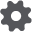# Hertz-Mindlin with JKR

Hertz-Mindlin with JKR (Johnson-Kendall-Roberts) Cohesion is a cohesion contact model that accounts for the influence of Van der Waals forces within the contact zone and allows the user to model strongly adhesive systems, such as dry powders or wet materials. In this model, the implementation of normal elastic contact force is based on the Johnson-Kendall-Roberts theory reported in (Johnson, Kendal and Roberts 1971).

Hertz-Mindlin with JKR Cohesion uses the same calculations as the Hertz-Mindlin (no slip) contact model for the following types of force:

1. Tangential elastic force.
2. Normal dissipation force.
3. Tangential dissipation force.

JKR normal force depends on the overlap δ and the interaction parameter, surface energy γ in the following way:Here, E* is equivalent Young’s modulus, and R* is the equivalent radius defined in the “Hertz-Mindlin (no slip) Contact Model” section.Normal force as a function of normal overlap. Hertz-Mindlin with JKR cohesion model results are compared with Hertz-Mindlin (no slip) model results. Negative overlap is the gap between two separated particles.

The EDEM JKR normal force follows the same solution of the above equations for both loading and unloading phases. Figure above shows the typical plot of JKR normal force as a function of normal overlap.

For γ = 0, force turns into Hertz-Mindlin normal forceThis model provides attractive cohesion forces even if the particles are not in physical contact. The maximum gap between particles with non-zero force is given byFor δ< δc the model returns zero force. The maximum value of the cohesion force occurs when particles are not in physical contact and the separation gap is less than δc. The value of maximum cohesion force, called pull-out force, is given byFriction force calculation is different than in the Hertz-Mindlin (no slip) contact model in that it depends on the positive repulsive part of JKR normal force. As a result, the EDEM JKR friction model provides higher friction force when cohesion component of the contact force is higher. The importance and advantages of this friction force model correction in the presence of strong cohesive forces was noted and illustrated in, for example, (Baran, et al. 2009), (Gilabert, Roux and Castellanos 2007).

Although this model was designed for fine, dry particles, it can be used to model wet particles. The force needed to separate two particles depends on the liquid surface tension γc and wetting angle θEquating the above force to JKR max forceallows JKR surface energy parameter estimation if EDEM particle size is not scaled.

 Interaction Configurable Parameters Position Particle to Particle,Particle to Geometry Click + to add cohesion to particle-particle or particle-geometry interactions. Set the surface energy for each interaction. Surface energy is a property of the materials ability to retain moisture/charge on its surface. The amount of surface energy influences the adhesion of the material. The SI units of surface energy are J/m². Last

Use:

1. Select the required category from the Interaction pulldown in the Physics section of the Creator.

2. Click the + drop-down list then select Hertz-Mindlin with JKR Cohesion.

3. Click the configuration buttonto define contact model parameters.

Note: It is not recommended to use this contact model with particle contact radius on since this will result in an attractive force before a physical contact has been made (see Hertz-Mindlin with JKR Version 2).